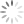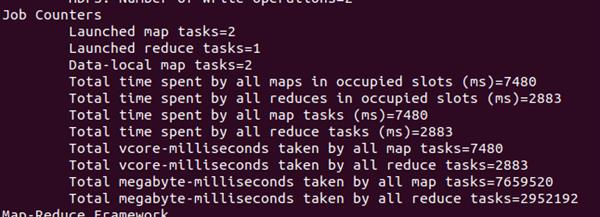Loading, please wait...# Evaluating execution time for multiplication of various multi-dimensional matrix in Hadoop.

Dec 11, 2019 Matrix Hadoop Big Data, Multiplication, 3477 Views
In This Article, we'll discuss A Evaluating execution time for multiplication of various multi-dimensional matrix in Hadoop

In Hadoop, Map Reduce is a processing function which is used to slenderize the task into two parts and these parts works in different way. MapReduce includes one mapper and one reducer. Various Map Reduce algorithms are used to handle huge amount of data that comes from different locations .Online data are in scattered form and stored in different memory locations as well as far from each others Mapper takes large set of data and converts it into another set of data in which every single element is  broken down into key/value pairs. On the other side, reduce takes the output from mapper as an input after that combines data tuples(key/values) into a smaller set of tuples. Matrix multiplication algorithm with mapreduce are used to compare the execution time complexity and space complexity. In this paper we took different sizes of matrix and calculate the execution time according to their sizes on the Hadoop environment.

### I. Introduction

Big data is used to refer to data sets that are too large or complex for traditional data-processing application software to adequately deal with. In other words, Big data usually includes data sets with sizes beyond the ability of commonly used software tools to capture, curate, manage, and process data within a tolerable elapsed time.Big data deals with unstructured, semi-structured and structured data, however the main focus is on unstructured data. The data is generated rapidly and continuously. When we want to know about something we use search engines, when we want to socialize we use social sites like facebook, twitter, Instagram etc which in turn generates a huge amount of data. And when a toddler wants to listen to the poems or wants to watch cartoons he just opens youtube and watches it. Even a single click by the user generates data and it is generated 24*7. We generate data in PB’s on daily basis.

### II. Characteristics of big data

Big data consists of the following characteristics:

Volume-It means the quantity of generated and stored data. The size of the data helps us to determines the value and potential insight, and whether it can be considered as big data or not.

Variety-It means what is the type and nature of the data. It may be used to help people who analyze it to effectively use the resulting insight.It draws from text, images, audio, videos and also it completes missing pieces through data fusion.

Velocity-It basically refers to the speed at which the data is generated and processed to meet the demands and challenges that lie in the path of growth and development. Big data is often available in real-time.

Veracity-It refers to the accuracy of the data .it is used to analyze whether the data is adequate or not.

• APPLICATIONS
• Government
• Internet of Things
• Healthcare
• International development
• E-commerce
• Education
• Media

III. CHALLENGES

Scalability-While working with  big data, it’s very important to be able to scale up and down on-demand. Many of the organizations fail to account how quickly a big data project can grow and expand.

Security-Keeping this large amount of data secure is another great challenge. Some of challenges include:

• To perform the user authentication for every team and team member accessing the data.
• To record each data access histories and meeting other consent regulations
• To properly make the use of encryption on data in-transit and at rest.

Syncing Across Data Sources-Once we import our data into big data platforms we may realize that data copies migrated from a wide range of sources on different rates and schedules can rapidly get out of the synchronization with the originating system.

Miscellaneous Challenges-Other challenges can be occured while we try to integratethe large amount of data. These challenges may include integration of data, skill availability, solution cost, the volume of data, the speed of transformation of data, veracity and validity of data.

IV. MATRIX MULTIPLICATION IN BIG DATA

Matrix multiplication in Big Data refers to the process in which we have two matrices as input and produces one matrix as an output by performing multiplication operation on them using Map-Reduce.Matrix Multiplication can also be applied in Business field also. Such as, Market shops , Shopping malls , Online Shopping sites etc. Top Companies like Amazon , IBM , SnapDeal , Flipcart etc; are using it.

Through matrix multiplication, a Businessman can determine the most purchased product in the market or the effect of purchase quantitydue to discount day based on the amount of purchase. Different matrix multiplication methods can be implemented on marketthat uses matrices for the cost ofeach product and the quantity purchased for each product. They can also use it to know the relation between their products.

V. HOW MAP-REDUCE WORKS IN MATRIX MULTIPLICATION

Matices normally consists of large amount of data . In this we can utilize data blocks to balance processing overhead results from a small mapper set and I/O overhead results from a large mapper set. Balancing between these two processing steps, consumes a lot of time and resources. Matrix-Mltiplication uses single MapReduce job and pre- processing step. In the pre-processing step it reads an element from the first array and a block from the second array prior to merging both elements into one file. After that the map task performs the multiplication operations, whereas the reduce task performs the sum operations.

MapReduce enables efficient parallel and distributed computing and consists of two serial tasks that are: Map and Reduce as discussed above paragraph. Each of the task is implemented with several parallel sub-tasks. Map task is the first task in MapReduce which accepts the input for conversion into a different form as the output. In this task, both the input and the output data are generated using a series of elements with individual key-value pairs. The reduce task takes the output of map task as an input and implements an aggregation process for pairs with identical keys.

MATRIX MULTIPLICATION MAPPING FUNCTION-

``````public class Map  extends

<Long, Text, Text, Text>

{

public void map(Long key, Text value, Context context)

throws

IOException, Interrupted Exception

{

Configuration conf = context.getConfiguration();

int m = Integer.parseInt(conf.get("m"));

int p = Integer.parseInt(conf.get("p"));

String line = value.toString();

String[] indicesAndValue = line.split(",");

Text outputKey = new Text();

Text outputValue = new Text();

if (indicesAndValue.equals("M"))

{

for ( k< p)

{

outputKey.set (indicesAndValue + "," + k);

outputValue.set(indicesAndValue + "," + indicesAndValue + "," +

indicesAndValue);

context.write();

}

} else {

for (int i = 0; i < m; i++) {

outputKey.set(i);

outputValue.set(N);

context.write(outputKey, outputValue);

}}}}

``````

MATRIX MULTIPLICATION REDUCING FUNCTION:-

``````public class Reduce

extends org.apache.hadoop.mapreduce.Reducer<Text, Text, Text, Text> {

public void reduce(Text key, Iterable<Text> values, Context context)

throws IOException, InterruptedException {

String[] value;

HashMap<Integer, Float> hashA = new HashMap<Integer, Float>();

HashMap<Integer, Float> hashB = new HashMap<Integer, Float>();

for (Text val : values) {

value = val.toString().split(",");

if (value.equals("M")) {

hashA.put(Integer.parseInt(value), Float.parseFloat(value));

} else {

hashB.put(Integer.parseInt(value), Float.parseFloat(value));

} }

int n = Integer.parseInt(context.getConfiguration().get("n"));

float result = 0.0f, m_ij, n_jk;

for (int j = 0; j < n; j++) {

m_ij = hashA.containsKey(j) ? hashA.get(j) :0.0f;

n_jk = hashB.containsKey(j) ? hashB.get(j) : 0.0f;

result += m_ij * n_jk; }

if (result != 0.0f) {

context.write(null, new Text(key.toString() + "," + Float.toString(result)));

}}}``````

EXAMPLE

Suppose we have two Matrices i.e Matrix A and Matrix B:

As input data files, we store Matrix A and B on Hdfs in this format:For matrix A,map task will produce Key and value pairs:

(I, k)(A,j,Aij)

A11=1

(1,1),(A,1,1) k=1

(1,2),(A,1,1) k=2

A12=2………..up to no. of elements in B

For second Matrix, map task will produce the pairs as :

(I,k)(B,j,Bjk)

B11=a

(1,1)(B,1,a) I =1

(2,1)(B,1,a) I =2………………upto no. of elements in A

Now the combine operation will be executed. After this map will return the key,valuepair for each element of both the matrices (all the possible values of j)

((I,k),[(A,j,Aij),(A,j,Aij),………..(N,j,njk),(N,j,njk),…..])

The entries for same key will be saved in the same list and stored in hdfs. Then the output of Map task will be given to Reduce as input.

Now the reducer will process each key at a time.for each key (I,k) it divides the values in 2 separate lists for A and B.for key (1,1), the value is the list of[(A,1,1),(A2,2),(A,3,3),(B,1,a)(B,2,c)(B,3,e)]

After that, the reducer will sort the values begin with A by j in one list and with B by j in the other list. Then it will sum up the multiplication of Aij and Bjk for each j. The same computation is applied to all input entries to reducer.The product matrix M of AB is then generated with execution time and occupied slots of map and reduce as:

Result:-